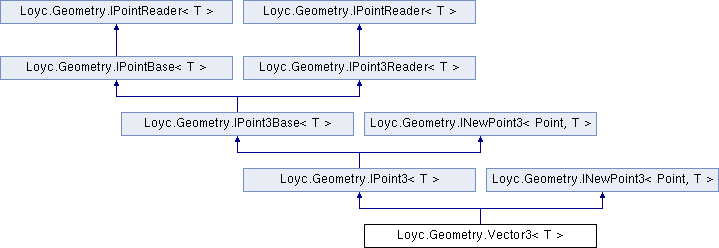Enhanced C# Language of your choice: library documentation

# Documentation moved to ecsharp.net

GitHub doesn't support HTTP redirects, so you'll be redirected in 3 seconds.

Loyc.Geometry.Vector3< T > Struct Template Reference

Represents a three-dimensional vector, i.e. a magnitude and direction or the difference between two points, stored as X, Y and Z components. More...

Source file:
Inheritance diagram for Loyc.Geometry.Vector3< T >:## Remarks

Represents a three-dimensional vector, i.e. a magnitude and direction or the difference between two points, stored as X, Y and Z components.

A vector is the same as a point except for the operations it supports. For example, it usually does not make sense to add two points, but you can add two vectors (to get another vector) or you can add a vector to a point (to get a point).

If you really do need to add two points together or something like that, you can typecast from Point3{T} to Vector3{T}.

Loyc.Geometry.PointMath, Loyc.Geometry.VectorMath{T}, Point3{T}
Type Constraints
 T : IConvertible T : IEquatable

_y

_z

## Public static fields

static ISignedMath< T > m = Maths<T>.SignedMath

static readonly Vector3< T > Zero = new Vector3<T>()

static readonly Vector3< T > Inf = new Vector3<T>(m.PositiveInfinity, m.PositiveInfinity, m.PositiveInfinity)

## Properties

X` [get, set]`

Y` [get, set]`

Z` [get, set]`Properties inherited from Loyc.Geometry.IPoint3Base< T >
new T Z` [get, set]`
Z coordinate of a point or vector. More...Properties inherited from Loyc.Geometry.IPointBase< T >
new T X` [get, set]`
Horizontal coordinate of a point or vector. More...

new T Y` [get, set]`
Vertical coordinate of a point or vector. More...Properties inherited from Loyc.Geometry.IPointReader< T >
X` [get]`

Y` [get]`Properties inherited from Loyc.Geometry.IPoint3Reader< T >
Z` [get]`

## Public Member Functions

Vector3 (T x, T y)

Vector3 (T x, T y, T z)

override bool Equals (object other)

override int GetHashCode ()

override string ToString ()

Vector3< T > New (T x, T y, T z)

IPoint3< T > INewPoint3
< IPoint3< T >, T >.
New (T x, T y, T z)

bool Equals (Vector3< T > other)

## Static Public Member Functions

static operator Point3< T > (Vector3< T > p)

static operator Vector3< int > (Vector3< T > p)

static operator Vector3< long > (Vector3< T > p)

static operator Vector3< float > (Vector3< T > p)

static operator Vector3< double > (Vector3< T > p)

static Vector3< T > operator+ (Vector3< T > a, Vector3< T > b)

static Vector3< T > operator- (Vector3< T > a, Vector3< T > b)

static Vector3< T > operator* (Vector3< T > p, T factor)

static Vector3< T > operator/ (Vector3< T > p, T factor)

static Vector3< T > operator<< (Vector3< T > p, int amt)

static Vector3< T > operator>> (Vector3< T > p, int amt)

static T operator* (Vector3< T > a, Vector3< T > b)
Dot product. a*b equals lhs.Length*rhs.Length*Cos(theta) if theta is the angle between two vectors. More...

static Vector3< T > operator- (Vector3< T > a)
Reverses a vector. More...

static bool operator== (Vector3< T > a, Vector3< T > b)

static bool operator!= (Vector3< T > a, Vector3< T > b)

## Member Function Documentation

 static T Loyc.Geometry.Vector3< T >.operator* ( Vector3< T > a, Vector3< T > b )
inlinestatic

Dot product. a*b equals lhs.Length*rhs.Length*Cos(theta) if theta is the angle between two vectors.

 static Vector3 Loyc.Geometry.Vector3< T >.operator- ( Vector3< T > a )
inlinestatic

Reverses a vector.